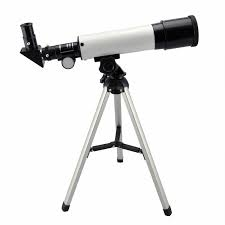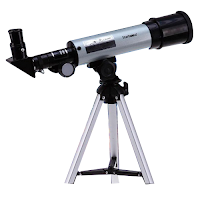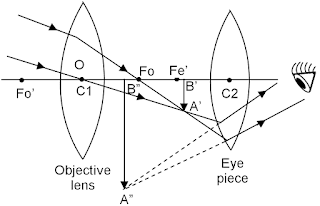# Different types of telescope class 12

Have you seen those big laboratories with such a huge lens so that you can observe stars and other planets ? Well, they are nothing but just big telescopes that are used to observer outer space and have a close look at the stars and planet that we can't see with our naked eye.

So, in this article, we are going to have a closer look at the telescope or to be precise types of the telescope which are in class 12th.

A telescope is a device used to see a distant object which we can't see by our naked eye. There are generally two types of telescope 1. Terrestrial telescopes and 2. Astronomical telescope which is of great importance in astronomy.

## Types of telescope:

As I said earlier, in this article we are going to focus on only two types of a telescope which are in your class 12th syllabus.  1. Terrestrial telescope and 2. Astronomical telescope.

Both these telescopes lie under the same category as the refracting telescope. A refracting telescope has two convex lenses, the larger one is called the objective lens whereas the smaller one is for viewing called the eyepiece.

Now both these telescope sounds almost identical and therefore it may lead to many confusion so here are the basic difference between the terrestrial telescope and astronomical telescope to give you guys a brief idea about it ;)

Difference between a terrestrial and astronomical telescope
S.no Terrestrial Astronomical
1. Used to observe things on the ground. Used to observe astro-objects such as
stars, moons, etc..
2. Designed for magnification. Designed to gather more light.
3. Terrestrial telescope presents an upright image. Astronomical telescope presents an upside down image
and requires an "erecting prism" between scope and eyepiece to correct it.

## Terrestrial telescope:

As mentioned in the table above, Terrestrial telescopes come in the category of a refracting telescope which consists of three lenses. This telescope is used to see distant objects on earth.A terrestrial telescope.

### Working and ray diagram:

In optics (Study of light), a ray diagram is a way to understand the path of light and how it travels after getting reflected or refracted through a lens or mirror.

P.s: If you don't know, what reflection or refraction is then we have a different article where we have covered this. Click here to read that article.

This ray diagrams also helps us to understand the mechanism of the terrestrial telescope, here is the ray diagram of a terrestrial telescope.Ray diagram of a terrestrial telescope

Unlike other refracting telescopes, the terrestrial telescope consists of 1 extra lens known as the erecting lens, in total, a terrestrial telescope consists of three lenses.

Now, if you look in the above ray diagram, the terrestrial telescope consists of three lenses, the biggest lens is known as the objective lens used to gather light and the 2nd largest lens which is used for viewing is known as eyepiece.

After light passes from the objective lens, it forms a reversed image which doesn't matter if you are seeing an astronomical object such as stars but the terrestrial telescope is used to see distant objects on earth and therefore we need to get an erect image (straight image) and therefore an erecting lens is used (smallest of the three).

## Astronomical telescope:

Astronomical telescope is also a refracting telescope used to see distant astro objects such as moons, stars, etc.. Unlike terrestrial telescope, the astronomical telescope consists of only two lenses and doesn't have an erecting lens.An Astronomical telescope

### Working and ray diagram:

To understand the working of astronomical telescope, we need to look at the ray diagram (path of light) in an astronomical telescope.Ray diagram of an astronomical telescope.

Now, the astronomical telescope consists of only two lenses and doesn't have an "erecting lens" like a terrestrial telescope.

This ray diagram shows the working of astronomical telescope, light from the astro body such as stars, the moon falls on the objective lens (bigger one in ray diagram) and forms an inverted real image A'B. This image A'B' now acts as an object for eyepiece lens (smaller one in ray diagram) and an image is formed (A''B'') at infinity if you look it through eyepiece lens.

One thing to note here is, an image formed by the astronomical telescope is always inverted because we don't use an "erecting lens" here and it doesn't even matter whether image formed is inverted or erected because all the astro bodies are mostly spherical in shape and when you are dealing with an object which is that far then shape actually doesn't matter that much.

## Notes:

Telescope comes under the optics or to be precise ray optics. I already have another article on this site where I have embedded and provided my personal notes of Ray Optics.

Here is the link of that article if you want my personal notes of Ray Optics (Notes cover all the topics of Ray Optics of class 12th including telescope)

## Conclusion:

So this was all about the telescope we are going to learn in class 12th. 1. Terrestrial telescope 2. Astronomical telescope.

Both these telescope are refractive telescope as lightly used to get refracted from the lens. It's not that, there are only these two types of telescope out there, even astronomical telescope as many variants and types but that's not the topic of this article and its not even in class 12th.

I hope all your queries are cleared, if not feel free to comment down below. I will more than happy to help you guys :).